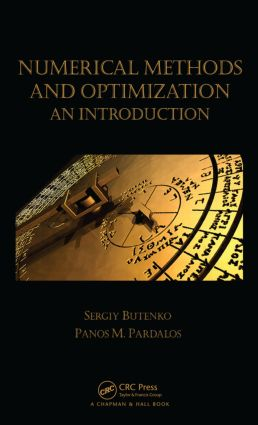# Numerical Methods and Optimization

## An Introduction, 1st Edition

Chapman and Hall/CRC

412 pages | 53 B/W Illus.

##### Purchasing Options:\$ = USD
Hardback: 9781466577770
pub: 2014-03-11
SAVE ~\$18.99
\$94.95
\$75.96
x
eBook (VitalSource) : 9780429188428
pub: 2014-03-11
from \$47.48

FREE Standard Shipping!

### Description

For students in industrial and systems engineering (ISE) and operations research (OR) to understand optimization at an advanced level, they must first grasp the analysis of algorithms, computational complexity, and other concepts and modern developments in numerical methods. Satisfying this prerequisite, Numerical Methods and Optimization: An Introduction combines the materials from introductory numerical methods and introductory optimization courses into a single text. This classroom-tested approach enriches a standard numerical methods syllabus with optional chapters on numerical optimization and provides a valuable numerical methods background for students taking an introductory OR or optimization course.

The first part of the text introduces the necessary mathematical background, the digital representation of numbers, and different types of errors associated with numerical methods. The second part explains how to solve typical problems using numerical methods. Focusing on optimization methods, the final part presents basic theory and algorithms for linear and nonlinear optimization.

The book assumes minimal prior knowledge of the topics. Taking a rigorous yet accessible approach to the material, it includes some mathematical proofs as samples of rigorous analysis but in most cases, uses only examples to illustrate the concepts. While the authors provide a MATLAB® guide and code available for download, the book can be used with other software packages.

### Reviews

"The book is in most parts very well developed and is served by nice illustrations, a fluid style of writing, and a layout that makes it easy to read. … [it will] serve well its purpose of bridging the gap between numerical analysis, operations research, and mathematical optimization for undergraduate students in the applied sciences."

Mathematical Reviews, August 2014

"If you are looking for an enjoyable and useful introduction to the basic topics of numerical methods and optimization, this is the right text to read. The authors are not only experienced lecturers but also active researchers in this area. They present the basic topics of numerical methods and optimization in an easy-to-follow, yet rigorous manner. In particular, they gently introduce some important topics, such as computational complexity, which are usually unavailable in textbooks on optimization for engineers. The authors occasionally turn to mathematical humor (such as ‘There are 10 types of people—those who understand binary, and those who don't’) to illustrate some material in the text. This informal contact with the reader exemplifies the engaging style of exposition characteristic of this excellent book."

—Oleg Burdakov, Linkoeping University, Sweden

### Table of Contents

Basics

Preliminaries

Sets and Functions

Fundamental Theorem of Algebra

Vectors and Linear (Vector) Spaces

Matrices and Their Properties

Preliminaries from Real and Functional Analysis

Numbers and Errors

Conversion between Different Number Systems

Floating Point Representation of Numbers

Definitions of Errors

Round-off Errors

Numerical Methods for Standard Problems

Elements of Numerical Linear Algebra

Direct Methods for Solving Systems of Linear Equations

Iterative Methods for Solving Systems of Linear Equations

Overdetermined Systems and Least Squares Solution

Stability of a Problem

Computing Eigenvalues and Eigenvectors

Solving Equations

Fixed Point Method

Bracketing Methods

Newton’s Method

Secant Method

Solution of Nonlinear Systems

Polynomial Interpolation

Forms of Polynomials

Polynomial Interpolation Methods

Theoretical Error of Interpolation and Chebyshev Polynomials

Numerical Integration

Trapezoidal Rule

Simpson's Rule

Precision and Error of Approximation

Composite Rules

Using Integrals to Approximate Sums

Numerical Solution of Differential Equations

Solution of a Differential Equation

Taylor Series and Picard’s Methods

Euler's Method

Runge-Kutta Methods

Systems of Differential Equations

Higher-Order Differential Equations

Introduction to Optimization

Basic Concepts

Formulating an Optimization Problem

Mathematical Description

Local and Global Optimality

Existence of an Optimal Solution

Level Sets and Gradients

Convex Sets, Functions, and Problems

Complexity Issues

Algorithms and Complexity

Average Running Time

Randomized Algorithms

Basics of Computational Complexity Theory

Complexity of Local Optimization

Optimal Methods for Nonlinear Optimization

Introduction to Linear Programming

Formulating a Linear Programming Model

Examples of LP Models

Practical Implications of Using LP Models

Solving Two-Variable LPs Graphically

Classification of LPs

The Simplex Method for Linear Programming

The Standard Form of LP

The Simplex Method

Geometry of the Simplex Method

The Simplex Method for a General LP

The Fundamental Theorem of LP

The Revised Simplex Method

Complexity of the Simplex Method

Duality and Sensitivity Analysis in Linear Programming

Defining the Dual LP

Weak Duality and the Duality Theorem

Extracting an Optimal Solution of the Dual LP from an Optimal Tableau of the Primal LP

Correspondence between the Primal and Dual LP Types

Complementary Slackness

Economic Interpretation of the Dual LP

Sensitivity Analysis

Unconstrained Optimization

Optimality Conditions

Optimization Problems with a Single Variable

Algorithmic Strategies for Unconstrained Optimization

Method of Steepest Descent

Newton’s Method

Conjugate Direction Method

Quasi-Newton Methods

Inexact Line Search

Constrained Optimization

Optimality Conditions

Duality

Projected Gradient Methods

Sequential Unconstrained Minimization

Notes and References

Bibliography

Index

### Subject Categories

##### BISAC Subject Codes/Headings:
BUS049000
BUSINESS & ECONOMICS / Operations Research
MAT021000
MATHEMATICS / Number Systems
TEC029000
TECHNOLOGY & ENGINEERING / Operations Research